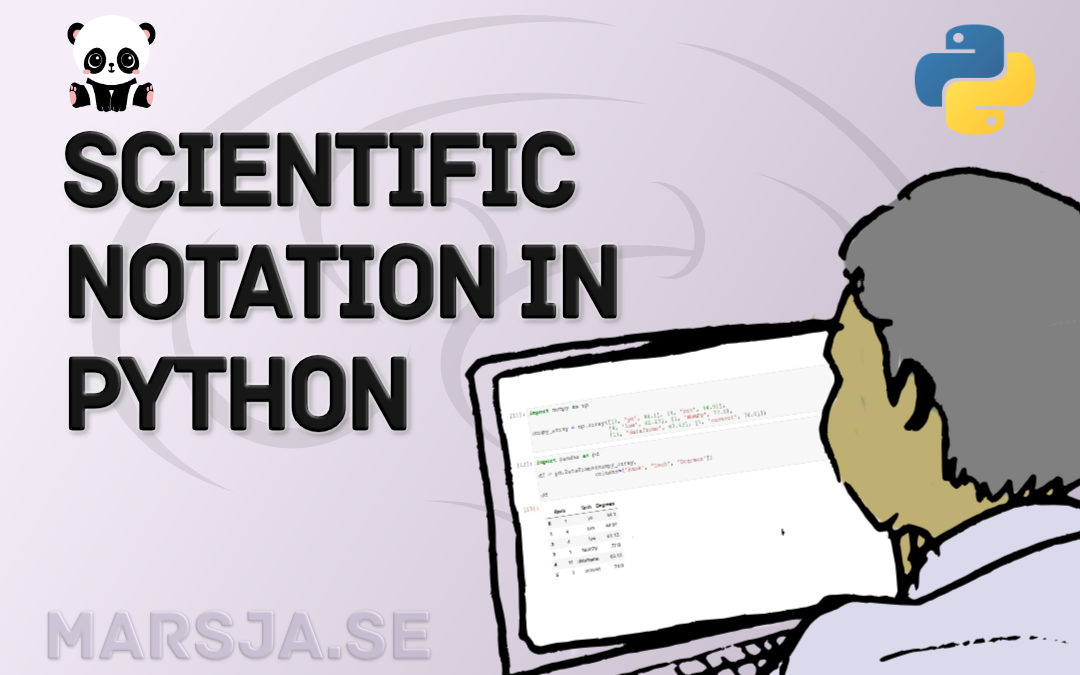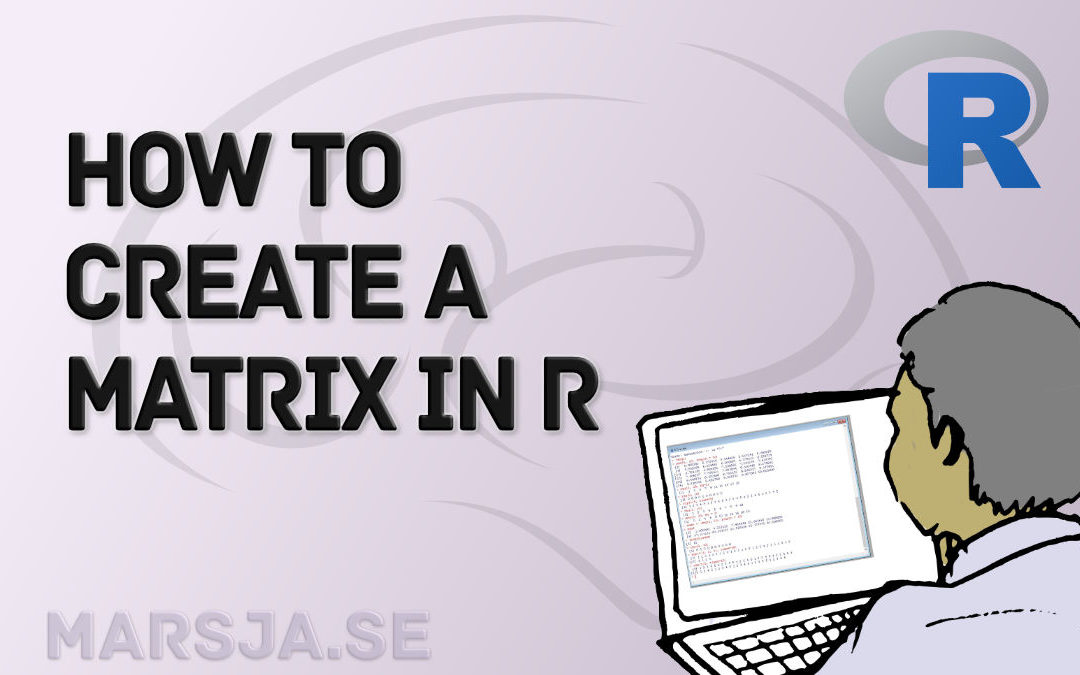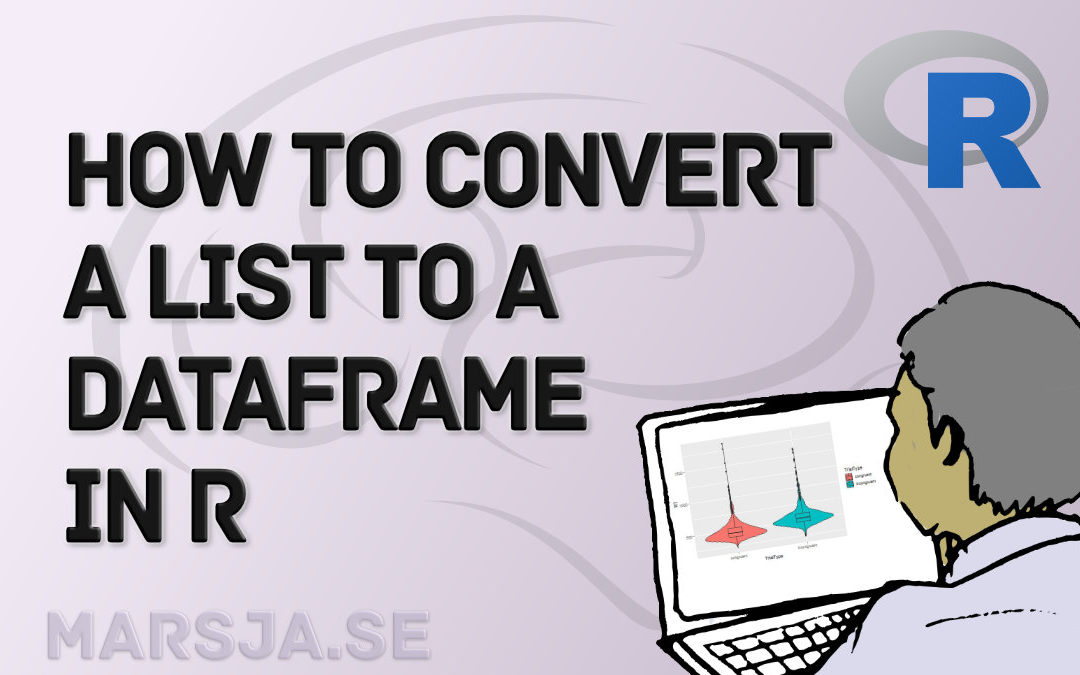In this PsychoPy tutorial, you will learn how to create the Psychomotor Vigilance Task (PVT) using some but not that much coding. Note, however, as the setup for this test has a random interstimulus interval (ISI), we will create a Psychomotor Vigilance Test with a...## How to Remove/Delete a Row in R – Rows with NA, Conditions, Duplicated

In this tutorial, you will 1) learn how to delete a row in R and 2) how to remove multiple rows in R. Of course, R being a versatile programming language, there are many different options when we need to delete a row. For example, we can use the subset() function if...## Python Scientific Notation & How to Suppress it in Pandas and NumPy

In Python, it is possible to print numbers in scientific notation using base functions as well as NumPy. Specifically, you will learn how to use Python to print very large or very small  (i.e., floating point) numbers in scientific notation using three different...## How to Create a Matrix in R with Examples – empty, zeros

In this short tutorial, you will learn how to create a matrix in R. We will use the matrix() function, among two other functions, for this aim. Specifically, we will go into the details of this function as this will enable us to e.g. name the columns and rows in the...## How to Convert a List to a Dataframe in R – dplyr

In this short tutorial, you will learn how to convert a list to a dataframe in R. To know how to convert lists to dataframes may be useful when you, for example, get your data from a source and they end up in a list of e.g. vectors. Here’s an example code template...